# 2nd PUC Maths Question Bank Chapter 6 Application of Derivatives Ex 6.4

Students can Download Maths Chapter 6 Application of Derivatives Ex 6.4 Questions and Answers, Notes Pdf, 2nd PUC Maths Question Bank with Answers helps you to revise the complete Karnataka State Board Syllabus and score more marks in your examinations.

## Karnataka 2nd PUC Maths Question Bank Chapter 6 Application of Derivatives Ex 6.4

### 2nd PUC Maths Application of Derivatives NCERT Text Book Questions and Answers Ex 6.4

Using differentials, find the approximate value of each of the following up to 3 places of decimal.

(i) $$\sqrt{25.3}$$(ii) $$\sqrt{49.5}$$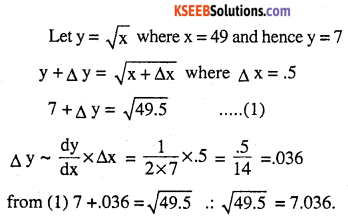(iii) $$\sqrt{0.6}$$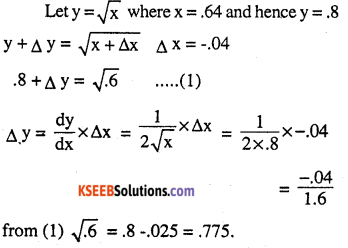(iv)$$(0.009)^{1 / 3}$$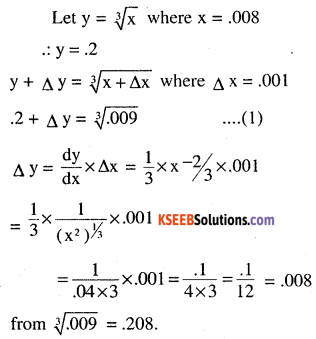(v) $$(0.009)^{1 / 3}$$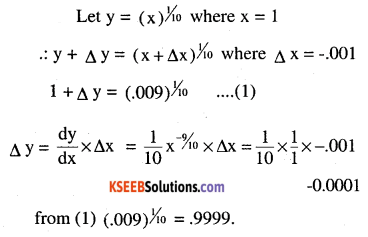(vi)$$(15)^{1 / 4}$$(vii)$$(26)^{1 / 3}$$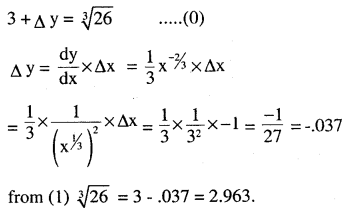(viii) $$(255)^{1 / 4}$$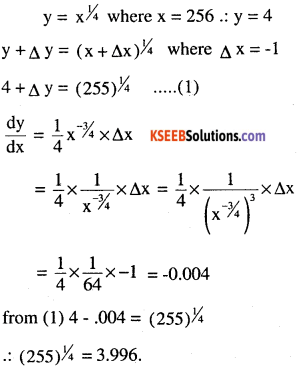(ix)$$(82)^{1 / 4}$$(x) $$(401)^{1 / 2}$$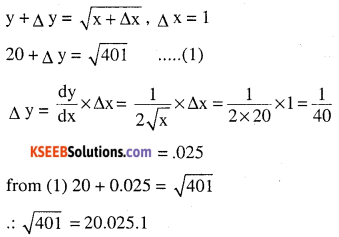(xi) $$(0.0037)^{1 / 2}$$(xii) $$(26.57)^{1 / 3}$$(xiii) $$(81.5)^{1 / 4}$$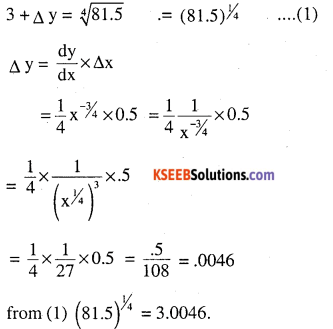(xiv)$$(3.968)^{3 / 2}$$(xv) $$(32.15)^{1 / 5}$$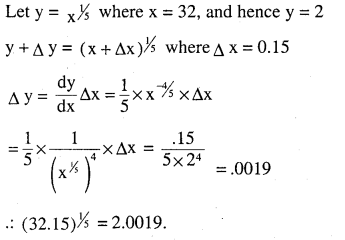Question 2.
Find the approximate value of f (2.01), where f (x) = 4x2 + 5x + 2.
f (x) = 4x2 + 5x + 2         .
f’ (x) = 8x + 5Question 3.
Find the approximate value of f (5.001), where f (x) = x3 – 7x2 + 15.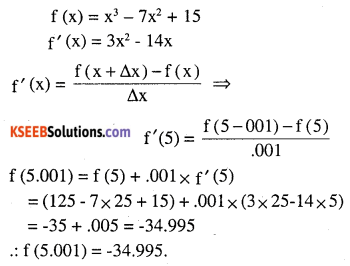Question 4.
Find the approximate change in the volume V of a cube of side x metres caused by increasing the side by 1%.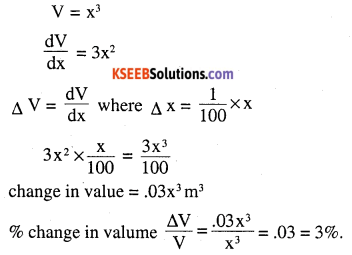Question 5.
Find the approximate change in the surface area of a cube of side x metres caused by decreasing the side by 1%.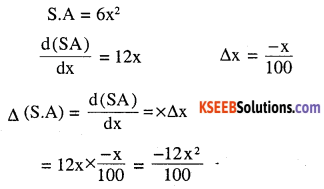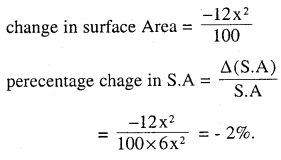Question 6.
If the radius of a sphere is measured as 7 m with an error of 02 m, then find the approximate error in calculating its volume.Question 7.
If the radius of a sphere is measured as 9 m with an error of 0.03 m, then find the approximate error in calculating its surface area.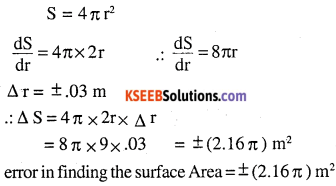Question 8.
If f(x) = 3x2 + 15x + 5, then the approximate value of f (3.02) is
(A) 47.66
(B) 57.66
(C) 67.66
(D) 77.66Question 9.
The approximate change in the volume of a cube of side x metres caused by increasing the side by 3% is
(A) 0.06 x3 m3
(B) 0.6 x3 m3
(C) 0.09 x3 m3
(D) 0.9 x3 m3